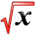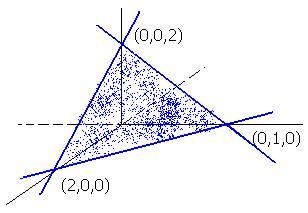# Graphing in 3DThis article is part of the MathHelp Tutoring Wiki

Ways to Graph in 3D

• Planes parallel to the plane xy have z = c (constant).
• Planes parallel to the plane xz have y = c (constant).
• Planes parallel to the plane yz have x = c (constant).
• Equation of plane is ax + by + cz + d = 0.

Example 1 :

Sketch the graph of z = -x-2y+2 = f(x,y).
Solution :
• Let z = 0 and you get y = -x/2 + 1.
If x = 0, then y = -0/2 + 1 = 1.
If y = 0, then x/2 = 1 therefore x = 2.
• Let x = 0 and you get z = -2y + 2.
If y = 0, then z = 2.
• Let y = 0 and you get z = -x + 2.

So the graph looks like this: